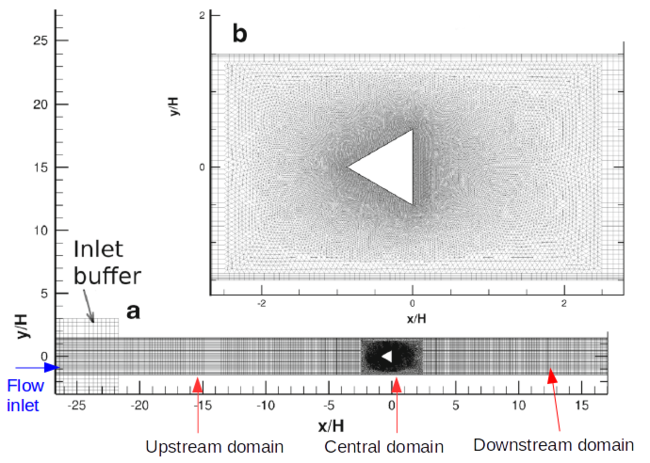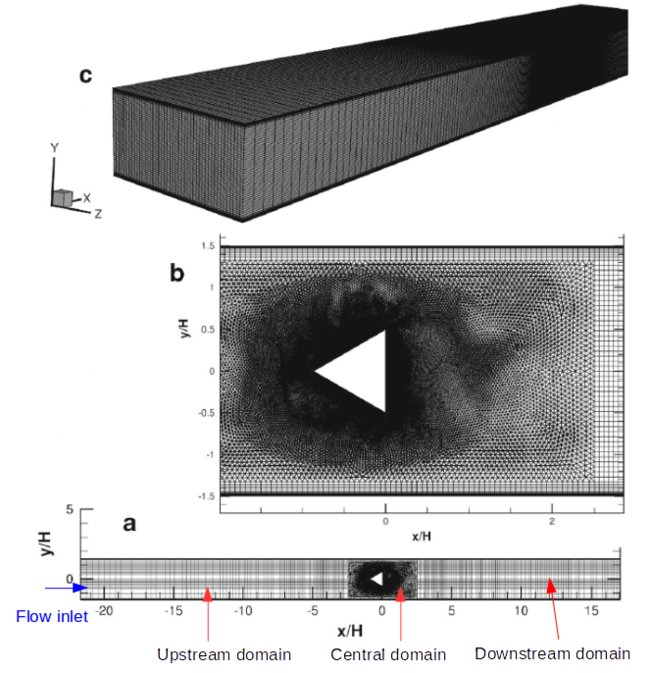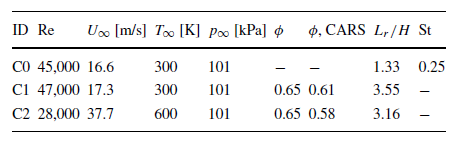CFD Simulations

Overview of CFD simulations

A series of numerical simulations was undertaken to study both inert and reactive flow over a the triangular bluff-body in order to replicate the experimental data and assess the different approaches and turbulence and combustion models [4,11]. The conventional Reynolds-averaged approach (RANS) with a family of the Launder-Sharma and realizable k-ε models has been used to calculate the non reactive flow. The details of the calculation approaches are described in [4,11].

A lean premixed propane/air bluff-body stabilized flame (Volvo test rig) was calculated using the Scale-Adaptive Simulation method (SAS) and Large-Eddy simulations (LES) as well as the conventional and unsteady Reynolds-averaged approach (RANS/URANS). RANS and SAS were closed by the standard k-ε and the k-ω Shear Stress Transport (SST) turbulence models, respectively. The conventional Smagorinsky and the k-equation sub-grid scale models were used for the LES closure. Effects of the sub-grid scalar flux modeling using the classical gradient hypothesis and Clark’s tensor diffusivity closures both for the inert and reactive LES flows are discussed. The Eddy Dissipation Concept (EDC) was used for the turbulence-chemistry interaction. Additionally, several RANS calculations were performed using the Turbulence Flame Speed Closure (TFC) model in Ansys Fluent to assess effects of the heat losses by modeling the conjugate heat transfer (hereafter CHT) between the bluff-body and the reactive flow. Also, the numerical results published by Ma et al.  and Jones et al.  are briefly discussed in the Best Practice Advice Section. Effects of the turbulent Schmidt number on the RAS results were discussed as well. Numerical results are compared with available experimental data. Reasonable consistency between experimental data and numerical results provided by RANS/URANS, SAS and LES was observed. In general, there is satisfactory agreement between present LES-EDC simulations, numerical results by other authors and measurements without requiring any major modification to the EDC closure constants, which gives a quite reasonable indication of the adequacy and accuracy of the method and its further application in turbulent premixed combustion simulations.

Effects of the turbulent Schmidt number on the RAS results were discussed as well. Numerical results are compared with available experimental data. Reasonable consistency between experimental data and numerical results provided by RANS/URANS, SAS and LES was observed. In general, there is satisfactory agreement between present LES-EDC simulations, numerical results by other authors and measurements without requiring any major modification to the EDC closure constants, which gives a quite reasonable indication of the adequacy and accuracy of the method and its further application in turbulent premixed combustion simulations.

Solution strategy

The calculations reported herein were performed using the finite-volume method implemented both in the commercial Ansys Fluent (AF hereafter)  and the open source OpenFOAM (OF hereafter)  (edcPisoFoam ) CFD codes. In the present study the RANS/URANS, SAS and LES approaches were used. It is worth noting that RANS results were obtained by AF, while URANS, SAS and LES results were obtained by OF.

Ansys Fluent

Using the factorized finite-volume method, the steady, incompressible Navier-Stokes equations were solved with a scheme-of second-order accuracy in space and time. The velocity and pressure fields were matched with a centered computational template based on the SIMPLEC algorithm within the spirit of Rhie and Chou. The convective terms were represented according to the second-order upwind scheme (SOU).

OpenFoam

The numerical method had second-order accuracy in space and time. The linear-upwind interpolation scheme (SOU) and linear (second-order central differences, CDS-2) interpolation were applied for approximating convective terms and other spatial derivatives, respectively, for the URANS) calculations. For the Scale-Adaptive and Large-Eddy simulations, the total variation diminishing (TVD) and normalized variable (NVD) schemes were used for the scalars to avoid unphysical overshoots and second law violations. A second-order implicit Euler method (BDF-2) was used for time integration together with the dynamic adjustable time stepping technique to guarantee a local Courant number less than 0.75 for URANS, SAS and LES.

To calculate the species reaction rate for each computational cell in the domain the robust LSODA algorithm  was used. Some results of the detailed validation and verification study of the new integrator were provided in Appendix A of Ref. . The relative tolerance, absolute tolerance and maximum number of iterations to meet the target accuracy were set to 10−5, 10−5 and 103, respectively.

Computational domain

The RANS/URANS grid

RANS/URANS calculations were performed for two-dimensional (2D) configurations only. The two-dimensional computational domain is presented in Fig. 7 and consisted of an inlet buffer domain (size of 0.2 m × 0.24 m) and a channel passage (size of 1.5 m × 0.12 m). It was decided to attach an inlet buffer domain to the main computational area, allowing the inlet velocity and temperature profiles to form implicitly during computations . The integration domain was split into three blocks to generate a high-quality unstructured quad/triangular mesh:

• the inlet buffer and a part of the channel without bluff-body. The channel part was resolved with 135 and 45 nodes in the horizontal and vertical directions, respectively, with exponential refinement of cells toward the bluff body;
• the central part of the channel passage with size of 0.2 m × 0.12 m, including the obstacle, as described in Fig. 7a. The bluff body sides contained 90 grid points, while the horizontal and vertical sides of the domain were resolved with 75 and 45 nodes, respectively, to obtain smooth mesh transition toward the flame holder;
• the remaining downstream part of the channel with resolution of 135 and 45 nodes in the horizontal and vertical directions, respectively, with exponential decreasing of nodes toward the outlet.

The viscous boundary layers were attached to the obstacle and the channel walls. The first element length, the growth factor and the total number of rows were set to 10−5 m, 1.2 and 11, respectively, for the triangular cylinder and 5×10−4 m, 1.2 and 5, respectively, for the channel walls. The distribution of the non-dimensional distance to the wall y* was about 1 both for the obstacle and the channel walls. Hereafter, this grid has label M1.Figure 7: General view of the computational domain for RANS/URANS (a), zoom of the grid (b) in the vicinity of the bluff-body. Here, x and y are the domain coordinates in stream-wise and transverse directions, according to the scheme in Fig. 2

The grid for SAS and LES

The computation domain and the unstructured mesh (hereafter, this grid has label M2) is shown in Fig. 8. The mesh was refined in the vicinity of the bluff body in order to resolve properly the separation of the laminar boundary layers. A total number of 2.2 million grid points were used in conjunction with three grid blocks. The integration domain was split into three blocks to generate the unstructured hexahedral/ tetrahedral mesh:

• the inlet channel without bluff-body. This channel part was resolved with 135×70×45 nodes in the stream-wise, transverse- and span-wise directions, respectively, with exponential refinement of cells toward the bluff body;
• the central part of the channel passage including the obstacle with size of

0.2 m × 0.12 m (Fig. 8b). The bluff body sides were discretized by 75 grid points, while the streamwise, transverse and span-wise lengths of the domain were resolved with 80×70×45 nodes, respectively, to obtain smooth mesh transition toward the flame holder;

• the remaining downstream part of the channel with resolution of 135×70×45 nodes

with exponential decreasing of nodes toward the outlet. The spanwise extent of the calculation domain was set to 6xH (same as in the experiments). The viscous boundary layers were attached to the obstacle and the channel walls. The first element length, the growth factor and the total number of rows were set to 10−4 m, 1.15 and 7, respectively, for the triangular cylinder and 10−4 m, 1.25 and 13, respectively, for the channel walls. The average distributions of the non-dimensional distance to the wall y0 were 4.6 and 5.4 for the obstacle and the channel walls, respectively.Figure 8: Details of the SAS and LES grid M2: description of the grid in x-y (a), zoom of the grid in x-y plane in the vicinity of the bluff-body (b) and general view of the grid (c). Here, x, y and z are the domain coordinates in stream-wise, transverse and span-wise directions, according to the scheme in Fig. 2

Grid dependence study

The present RANS/URANS results were obtained using two sets of grids: a low-resolution grid and a high resolution grid. The high-resolution grid was created by simple refinement of the low resolution grid by a factor of 2×2 in the horizontal and vertical directions as well as the flame holder. The details of the high-resolution grid were provided above. In general, the differences between the two sets of grids were insignificant (with 5% difference) for the mean velocity and temperature. The grid dependence study for the SAS and LES calculations was carried out using the refined grid (hereafter, M3). For this case, the M2 grid was simply adapted by a factor of 1.4 (the cell sizes were decreased by 1.4) leading to the total number of cells about of 4.7 M.

Boundary conditions

The inflow velocity, temperature and turbulence properties were specified based on the experimental settings. The inflow turbulence level was set to 3%. Constant values for inlet velocity and temperature (according to Table 5) were set for the RANS/URANS simulations. For the SAS and LES calculations the inlet velocity and temperature profiles were extracted and interpolated from the URANS predictions at the location x/H = −2. A zero-gradient pressure boundary was applied for all inflow boundaries, while the pressure at the outlet was specified to 101 kPa. The inlet values for the turbulence dissipation, ε, and specific dissipation, ω, were set according to the simple assumptions ε = Cμ0.75k1.5 1/Lm and ω = k0.5Cμ−0.25 1/Lm , where Cμ is the constant Cμ = 0.09 and Lm is the mixing length, which was set to the constant value Lm = 0.025 m. A no-slip condition for velocity was applied to the walls. The turbulence kinetic energy was set to the fixed small value of 10−12 m2/s2 at all walls, while the wall-functions for ε and ω  were used for the RANS/URANS and SAS models. The propane/air mixture was specified in terms of the species mass fractions. Zero-gradient diffusion boundary conditions were applied for species at the walls. Symmetry conditions were imposed at the lateral boundaries. Temperature boundary conditions at solid walls were treated according to the zero-gradient assumption. For RANS/URANS calculations also isothermal walls and the conjugate fluid-solid heat transfer were applied.

In the present SAS and LES simulations, the slip boundary condition was applied to the channel walls (which may be considered as a simplistic representation of flame quenching due to heat losses), since these calculations were focused on the model performance at the core central region of the flow. The reason for not adding perturbations at the inlet for SAS and LES calculations was: with the designed grid, the inflow perturbations would be highly damped due to grid expansion from the surface of the bluff-body toward the inlet boundary, and the probability that these perturbations would reach the triangular cylinder is small.

 Table 5: Flow parameters in calculations of Volvo rig cases: inert (C0) and reactive (C1-C2) casesComputational details (High performance computing)

OpenFOAM is a massively parallel open - source C++ class software based on message passing interface (MPI). The present calculations were carried out at the Vilje high performance computing (HPC) facility (https://www.sigma2.no/content/vilje), which is a distributed memory system that consists of 1440 nodes interconnected with a high bandwidth low-latency switch network (FDR Infiniband). Each node has two 8-core Intel Sandy Bridge (2.6 GHz) and 32 GB memory. Most present calculations were performed using 128 and 256 cores in parallel. The typical calculation time for one time step in reactive simulations varied between 10−20 s, and the total (effective) simulation time per run took around two weeks.

Contributed by: D.A. Lysenko and M. Donskov — 3DMSimtek AS, Sandnes, Norway